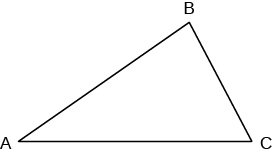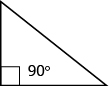## Key Concepts

• Supplementary and Complementary Angles
• If the sum of the measures of two angles is 180°, then the angles are supplementary.
• If angle $A$ and angle $B$ are supplementary, then $m\angle{A}+m\angle{B}=180$ .
• If the sum of the measures of two angles is $90^\circ$, then the angles are complementary.
• If angle $A$ and angle $B$ are complementary, then $m\angle{A}+m\angle{B}=90$ .
• Solve Geometry Applications
1. Read the problem and make sure you understand all the words and ideas. Draw a figure and label it with the given information.
2. Identify what you are looking for.
3. Name what you are looking for and choose a variable to represent it.
4. Translate into an equation by writing the appropriate formula or model for the situation. Substitute in the given information.
5. Solve the equation using good algebra techniques.
6. Check the answer in the problem and make sure it makes sense.
7. Answer the question with a complete sentence.
• Sum of the Measures of the Angles of a Triangle• For any $\Delta ABC$, the sum of the measures is $180^\circ$
• $m\angle{A}+m\angle{B}=180$
• Right Triangle• A right triangle is a triangle that has one $90°$ angle, which is often marked with a $\angle$ symbol.
• Properties of Similar Triangles
• If two triangles are similar, then their corresponding angle measures are equal and their corresponding side lengths have the same ratio.

## Glossary

angle
An angle is formed by two rays that share a common endpoint. Each ray is called a side of the angle.
complementary angles
If the sum of the measures of two angles is $90^\circ$ , then they are called complementary angles.
hypotenuse
The side of the triangle opposite the $90^\circ$ angle is called the hypotenuse.
legs of a right triangle
The sides of a right triangle adjacent to the right angle are called the legs.
right triangle
A right triangle is a triangle that has one $90^\circ$ angle.
similar figures
In geometry, if two figures have exactly the same shape but different sizes, we say they are similar figures.
supplementary angles
If the sum of the measures of two angles is $180^\circ$ , then they are called supplementary angles.
triangle
A triangle is a geometric figure with three sides and three angles.
vertex of an angle
When two rays meet to form an angle, the common endpoint is called the vertex of the angle.